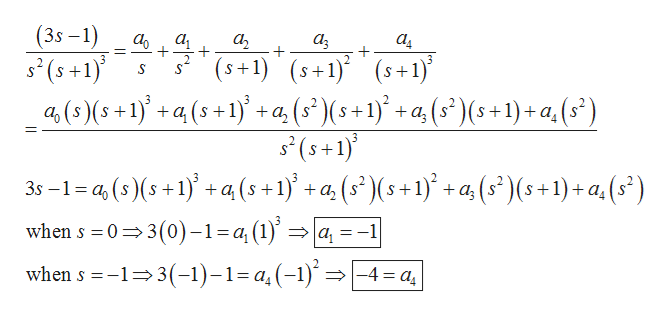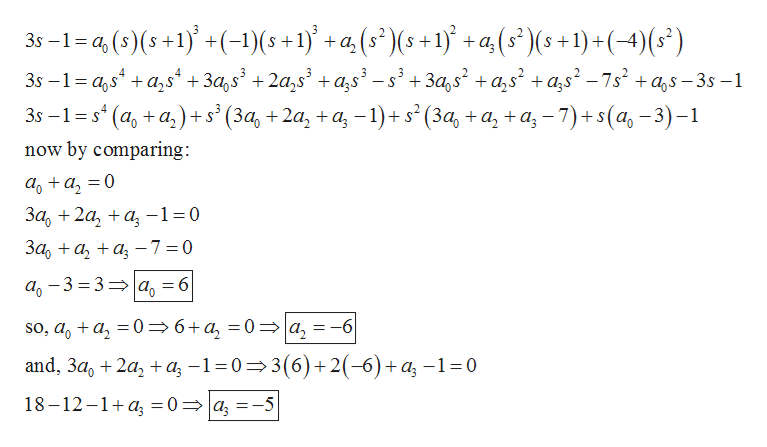Question
3 views

Find f(t).

−1 {(3s-1)/s2(s+1)3}

check_circle

Step 1

From the given information it is needed to calculate:

Step 2

Now, first take the partial fractions of the given expression and solve:help_outlineImage Transcriptionclose(3s -1 s2(s+1 4(9)(5 +1)} + q (s+1)' +a,(5* (5+1)' + 4,(s)(s+1)+ a, (s") а а а, а, + а, (s+1) (s+1)s+1) (s1) 3s-1-4(9)5+1) + a(s+1) + a, (s°)\( +1)* + a (5 )(5+1)+ a, (5") when s 0 3(0)-1 =a, (1) =a -1 when s 13(-)-1=a (-1) : -4 a fullscreen
Step 3

Now calculate rest of the unknowns by putting the v...help_outlineImage Transcriptionclose-1-4(9)(5+1+(-1)(s+1)+a(5')(5+1) +a, (s' )(s +1) +(-4)(s) S 3s- 1- а,s* +a,s* + За,s + 2а,s' +as - s + За,s+as' +a,s - 7s +as - 3s -1 35 -1%3s* (a, +a,)+s (За, + 2а, + а, -1) +s'(3а, +а, +а, -7)+s(a, - 3) -1 now by comparing aoa 0 За, + 2а, +а, -130 За, + а, + a, —73D0 а, - 3 %3 33D а, — 6 so, aa,0>6+a, = 0 a, = -6 and, 3a, 2aa -1 03(6)+2(-6)+ a -1 =0 18-12-1+ а, %3D0 %3D | а, 3-5 fullscreen

### Want to see the full answer?

See Solution

#### Want to see this answer and more?

Solutions are written by subject experts who are available 24/7. Questions are typically answered within 1 hour.*

See Solution
*Response times may vary by subject and question.
Tagged in

### Math